Mathguru

•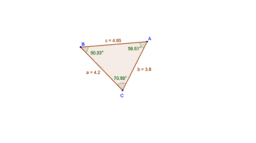The angle opposite to longest side is greatest

Activity

Mathguru

•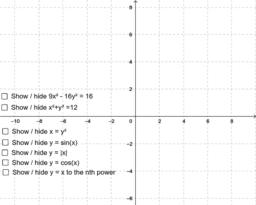Explore - Vertical Line Test

Activity

Mathguru

•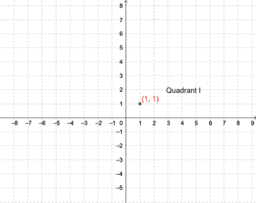Introduction to the coordinate plane

Activity

Mathguru

•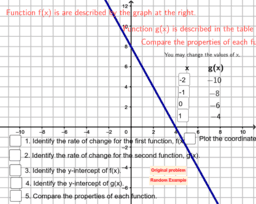Compare two linear functions

Activity

Mathguru

•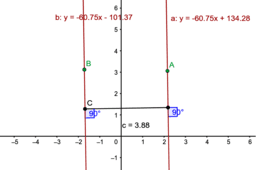distance between two parallel lines

Activity

Mathguru

•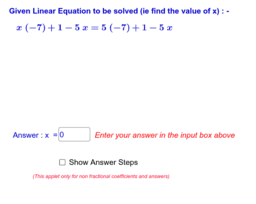Solve Simple Linear Equations (Apply Balance Concept)

Activity

Mathguru

•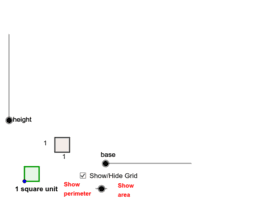Area and Perimeter of Rectangles

Activity

Mathguru

•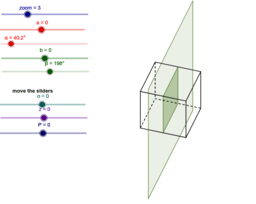cube cross section

Activity

Mathguru

•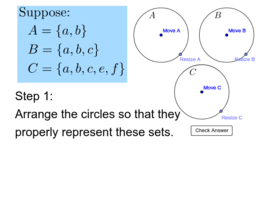Creating Diagrams of Subsets

Activity

Mathguru

•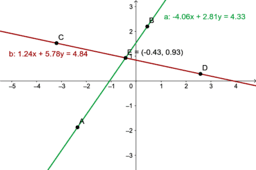solve a pair of linear equations in two variables.

Activity

Mathguru

•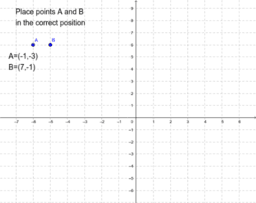Coordinate Points

Activity

Mathguru

•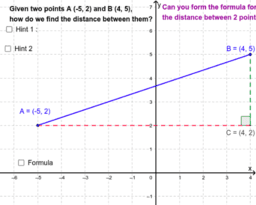Distance Between Two Points

Activity

Mathguru

•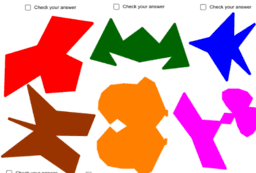Draw line of symmetry

Activity

Mathguru

•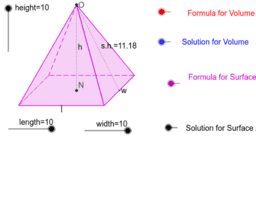Rectangular Pyramid

Activity

Mathguru

•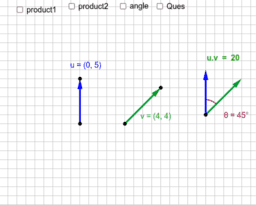Scalar product

Activity

Mathguru

•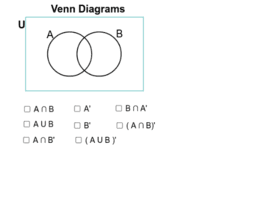Venn Diagrams

Activity

Mathguru

•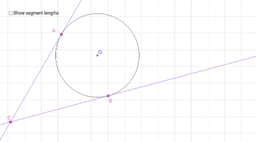Two Tangents Theorem

Activity

Mathguru

•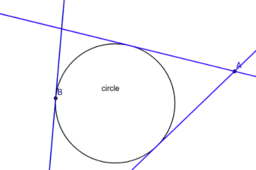number of tangents to a circle

Activity

Mathguru

•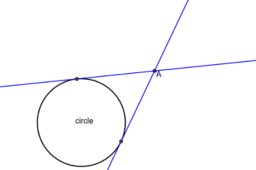The number of tangents drawn to a circle

Activity

Mathguru

•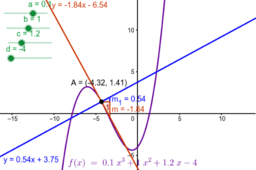Tangent and normal of a function using derivative

Activity

Mathguru

•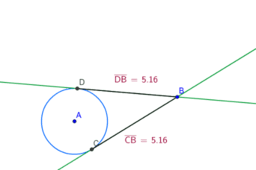length of tangents drawn from an external point to a circle

Activity

Mathguru

•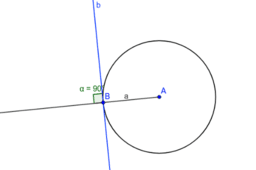Property of a tangent

Activity

Mathguru

•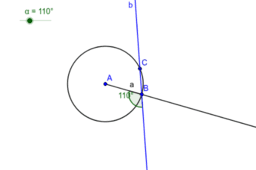Property of a tangent

Activity

Mathguru

•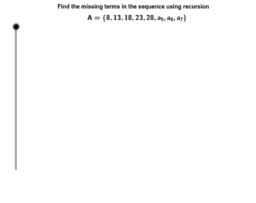Activity

Mathguru